search
Search
Unlock 100+ guides
search toc
close
account_circle
Profile
exit_to_app
Sign out
search
keyboard_voice
close
Searching Tips
Search for a recipe:
"Creating a table in MySQL"
Search for an API documentation: "@append"
Search for code: "!dataframe"
Apply a tag filter: "#python"
Useful Shortcuts
/ to open search panel
Esc to close search panel
to navigate between search results
d to clear all current filters
Enter to expand content previewDoc SearchCode Search BetaSORRY NOTHING FOUND!
mic
Start speaking...Voice search is only supported in Safari and Chrome.
Shrink
Navigate to
check_circle
Mark as learned
thumb_up
2
thumb_down
0
chat_bubble_outline
0
Comment
auto_stories Bi-column layout
settings

# Basics of set theory and Venn diagrams

schedule Aug 10, 2023
Last updated
local_offer
Tags

This guide will cover the essential basics of set theory that will be helpful when studying various fields of mathematics such as statistics, calculus and linear algebra.

# What are sets?

A set can simply be thought of as a list of elements where the ordering does not matter. For instance, the following is a set of some numbers:

$$A=\{1,3,\pi\}$$

Typically, an uppercase letter is used to denote a set. Sets don't necessarily have to hold numbers - they can hold virtually any element such as letters, variables and outcomes:

\begin{align*} C&=\{\text{h},\text{e},\text{l},\text{l},\text{o}\}\\ D&=\{d_1,d_2,d_3\}\\ E&=\{\text{heads},\text{tails}\}\\ \end{align*}

Since the ordering of the elements within a set does not matter, two sets are considered equivalent if they contain the same elements. For instance, the following two sets are equivalent:

$$\{1,2\}=\{2,1\}$$

# Special sets

The following are special sets that serve as the backbone of mathematics:

• the set $\mathbb{Z}=\{\cdots,-2,-1,0,1,2,\cdots\}$ is the set of all integers.

• the set $\mathbb{N}=\{1,2,3,\cdots\}$ is the set of all natural numbers.

• the set $\mathbb{Q}$ is the set of all rational numbers.

• the set $\mathbb{R}$ is the set of all real numbers.

• the set $\mathbb{C}$ is the set of all complex numbers.

To emphasize the fact that these are special sets, they are written in a font called blackboard bold - for instance, we write $\mathbb{R}$ instead of $R$.

## Empty sets

A set that contains no elements is called an empty set, represented by the symbol $\emptyset$.

## Universal sets

A universal set contains all the elements under consideration. For instance, suppose we roll a dice once - the universal set $S$ could represent all possible outcomes:

$$S=\{1,2,3,4,5,6\}$$

# Set notations

## Containment

To indicate that an element belongs to a set, we use the $\in$ symbol. For instance, consider the following set:

$$A=\{1,3,5\}$$

To state that $3$ is contained in this set $A$:

$$3\in{A}$$

In contrast, if an element does not belong to a set, then we use the $\notin$ symbol. For instance, to indicate that $4$ is not contained in our set $A$:

$$4\notin{A}$$

The containment symbol is extremely common in mathematics because we typically characterize the nature of a variable using $\in$. For instance, to indicate that a variable $x$ is an integer, we would write $x\in\mathbb{Z}$. In linear algebra, we denote a vector $\boldsymbol{v}$ in two-dimensional space as $\boldsymbol{v}\in\mathbb{R}^2$.

## Subsets

We say that set $A$ is a subset of set $B$ if and only if all elements in set $A$ are also contained in set $B$. For instance, consider the following sets $A$ and $B$ that contain some numbers:

\begin{align*} A&=\{1,2,4\}\\ B&=\{1,2,3,4,10\}\\ \end{align*}

Here, $A$ is a subset of $B$ because all the numbers within $A$ also belong to $B$. We can mathematically express this as follows:

$$A\subset{B} \;\;\;\;\text{or}\;\;\;\; B\supset{A}$$

Note that $A\subset{B}$ is to be read as "$A$ is a subset of $B$". Equivalently, we can call $B$ the superset of $A$, that is, $B$ contains all elements of $A$.

As another example, consider the set of natural numbers $\mathbb{N}$ and integers $\mathbb{Z}$:

$$\mathbb{N}\subset\mathbb{Z}$$

This means that the set of natural numbers is a subset of the set of integers.

## Intersection

The intersection of sets $A$ and $B$, which is denoted by $A\cap{B}$, is the set of all elements that are contained in both $A$ and $B$. For instance, consider the following set:

\begin{align*} A&=\{1,2,5\}\\ B&=\{1,2,3,9\}\\ \end{align*}

The numbers $1$ and $2$ both belong to sets $A$ and $B$. Therefore, the intersection of $A$ and $B$ is:

$$A\cap{B}=\{1,2\}$$

Notice how intersections are guaranteed to be subsets of both $A$ and $B$, that is:

$$(A\cap{B})\subset{A},\;\;\;\;\;\;\; (A\cap{B})\subset{B}$$

### Disjoint sets

Two sets $A$ and $B$ are considered disjoint sets if there is no common element between them. For instance, consider the following sets:

\begin{align*} A&=\{1,2,4\}\\ B&=\{3,5,6,7\}\\ \end{align*}

Because there exists no element that belongs to both sets $A$ and $B$, we say that $A$ and $B$ are disjoint sets. Mathematically, disjoint sets are expressed as:

$$A\cap{B}=\emptyset$$

This means that the intersection of $A$ and $B$ is the empty set.

## Union

The union of sets $A$ and $B$, which is denoted by $A\cup{B}$, is the combined set of elements in $A$ or $B$. For instance, consider the following sets:

\begin{align*} A&=\{1,2,4\}\\ B&=\{1,2,5\}\\ \end{align*}

The union of sets $A$ and $B$ is:

$$A\cup{B}=\{1,2,4,5\}$$

Notice how numbers $1$ and $2$ are contained in both $A$ and $B$, but they only appear once in the union. In other words, duplicates are removed in the resulting union.

## Exclusion

The operation $A\setminus{B}$ returns a set of elements in $A$ that do not exist in $B$. For instance, consider the following sets:

\begin{align*} A&=\{1,2,4\}\\ B&=\{1,4,5\}\\ C&=\{1,2\}\\ \end{align*}

To remove elements in $A$ and $B$ that belong to $C$, use the $\setminus$ operation like so:

\begin{align*} A\setminus{C}&=\{4\}\\ B\setminus{C}&=\{4,5\}\\ \end{align*}

## Cardinality

Cardinality of a set refers to the number of elements within a set. For instance, consider the following set:

$$A=\{1,2,4\}$$

The cardinality of $A$, denoted as $\vert{A}\vert$, is equal to 3.

NOTE

Since empty sets do not contain any elements, their cardinality is $0$, that is, $\vert{\emptyset}\vert=0$.

# Set-builder notation

Up to now, we have only defined sets explicitly like $A=\{1,2,3\}$. However, we can also define sets by specifying using the set-builder notation. For instance, let's define a set that contains even integers:

$$\begin{equation}\label{eq:ufvKee1gfATYcRyK2an} A=\{x\in{\mathbb{Z}}\;|\;x\text{ is even}\} \end{equation}$$

This is read as "the set of all integers $x$ such that $x$ is even". The vertical line $|$, which means "such that", is sometimes written as $:$ instead. The set-builder notation is quite flexible - we can also equivalently write \eqref{eq:ufvKee1gfATYcRyK2an} as follows:

$$\begin{equation}\label{eq:dV2gcJtkk9ewMjW95AC} A=\{x\;|\;x\in{\mathbb{Z}}\text{ and }x\text{ is even}\} \end{equation}$$

As another example, let's define another set that holds natural numbers less than $8$:

$$A=\{x\in{\mathbb{N}}\;|\;x\lt8\}$$

Finally, here's another one:

$$A=\{(x,y)\;|\;x\in\mathbb{R}\text{ and }y=x^2\}$$

This set contains all the points on a parabola!

# Intervals

## Closed intervals

The endpoints are included in the interval. These intervals are expressed using square brackets:

$$[a,b]=\{x\in\mathbb{R}\;|\;a\le{x}\le{b}\}$$

Here's an example:

$$[3,8]=\{x\in\mathbb{R}\;|\;3\le{x}\le{8}\}$$

The ends points $3$ and $8$ are included in this interval.

## Open intervals

The endpoints are not included in the interval. These intervals are expressed using parentheses:

$$(a,b)=\{x\in\mathbb{R}\;|\;a\lt{x}\lt{b}\}$$

The endpoints $a$ and $b$ are excluded from the interval.

## Mixing open and closed intervals

We could also mix the notation of open and closed intervals:

\begin{align*} [a,b)&=\{x\in\mathbb{R}\;|\;a\le{x}\lt{b}\}\\ (a,b]&=\{x\in\mathbb{R}\;|\;a\lt{x}\le{b}\} \end{align*}

## Denoting elements that belong to an interval

Suppose we wanted to mathematically state that a variable $x$ lies in the interval from $2$ to $6$ where both endpoints are included. We can use the containment symbol from earlier:

$$x\in[2,6]$$

This notation is extremely common in calculus!

# Venn diagrams to visualize sets

Venn diagrams are a useful way to visualize the relationship between sets. Typically, we use circles to represent sets, but we can in fact use any shape we wish. Consider the following set:

$$A=\{1,2,3\}$$

We can represent this set using a Venn diagram like so: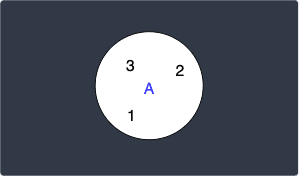We can think of the circle as the container of elements, just as the curly brackets $\{\cdots\}$ are in set notation.

## Intersection

Recall that the intersection of sets $A$ and $B$, which is denoted by $A\cap{B}$, is the set of all elements that are contained in both $A$ and $B$. For example, consider the following two sets:

\begin{equation}\label{eq:B4V9tinT2Y5LHf7E2Q7} \begin{aligned} A&=\{1,2,3\}\\ B&=\{3,4,5\}\\ \end{aligned} \end{equation}

The intersection $A\cap{B}$ can be visualized in a Venn diagram like so: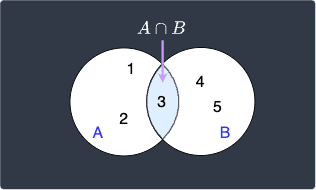Here, the shaded area represents the intersection of the two sets.

## Union

Recall that the union of sets $A$ and $B$, which is denoted by $A\cup{B}$, is the set of all elements that are contained in either $A$ or $B$. For example, consider the same sets \eqref{eq:B4V9tinT2Y5LHf7E2Q7} as above. The union of $A$ and $B$ can be visualized in a Venn diagram like so: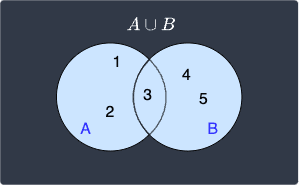Here, the shaded area in light blue represents the union of the two sets.

## Disjoint sets

Recall that sets $A$ and $B$ are called disjoint sets if they have no common elements. For example, consider the following two disjoint sets:

\begin{aligned} A&=\{1,2,3\}\\ B&=\{8,9\}\\ \end{aligned}

Disjoint sets are represented in a Venn diagram like so: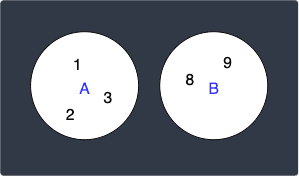Notice how unlike the previous Venn diagrams, the circles do not overlap, which means that the sets do not share any common element.

## Universal and complementary sets

Recall that the universal setlink contains all elements under consideration. For instance, suppose we roll a dice - the universal set $S$ could hold all possible outcomes:

$$S=\{1,2,3,4,5,6\}$$

Now, suppose we have a set $A$ that holds only the even outcomes:

$$A=\{2,4,6\}$$

The Venn diagram in this case would look like the following: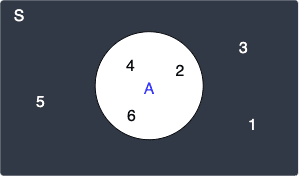The universal set $S$, as the name suggests, contains all the elements in the Venn diagram. Clearly, set $A$ is a subset of $S$.

A complementary set of $A$, which is denoted by $A'$ (or $A^c$ in some textbooks), holds all elements in the universal set $S$ that is not contained in $A$. This means that a complementary set contains everything else. In our dice example, the complementary set of $A$ will hold odd outcomes:

$$A'=\{1,3,5\}$$

In our Venn diagram above, the complementary set $A'$ is the set outside of $A$. To make this clearer, the light-blue region below represents $A'$: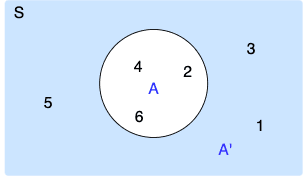Note that by definition, $A'$ will again be a subset of the universal set $S$.

thumb_up
thumb_down
Comment
Citation
Ask a question or leave a feedback...
thumb_up
2
thumb_down
0
chat_bubble_outline
0
settings
Enjoy our search
Hit / to insta-search docs and recipes!